# Math Brain Teasers 15:: Can You Find The Connection Between The Given Equations?

in STEMGeeks2 years ago

Hey All;

Its been a long time that we didn't have the Math Brain Teasers I guess we all needed a break and it did come along nicely. So here I'm with the Math Brain Teasers 15:: Can You Find The Connection Between The Given Equations?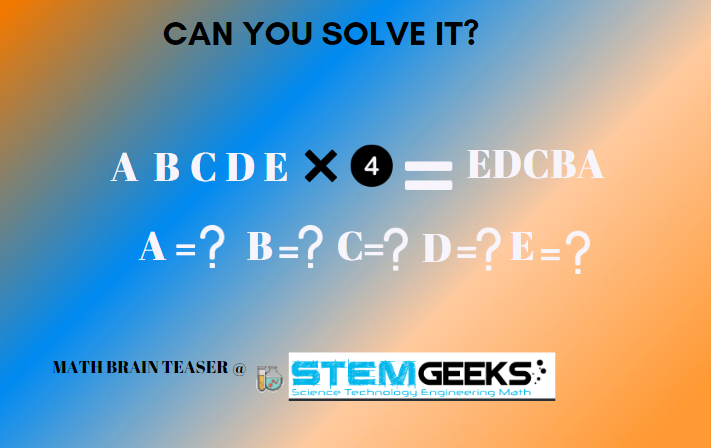Have a close look at the following equations in the given image and try finding out the pattern being followed and replace the question mark with your answer to the equation.

Hint:: First hint is that its not that complex equation to be solved. I assure you it's just a simple equation where A B C D & E are not Zero and they represent different numbers, so you just need to find them out; what numbers are they.

##### Math Brain Teasers 14:: How Many Numbers Do You See?? - Solved with Explanation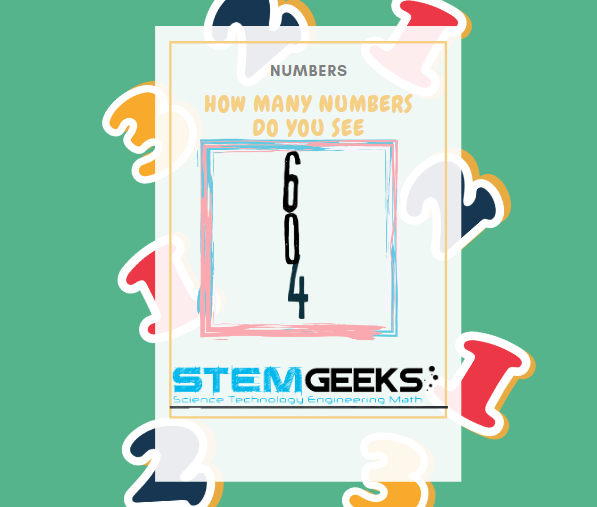The answer to:: How Many Numbers Do You See was 10 Numbers and the same can be depicted in the following image; which showcases how numbers from::

### 0 to 9 can be formed with the number "604""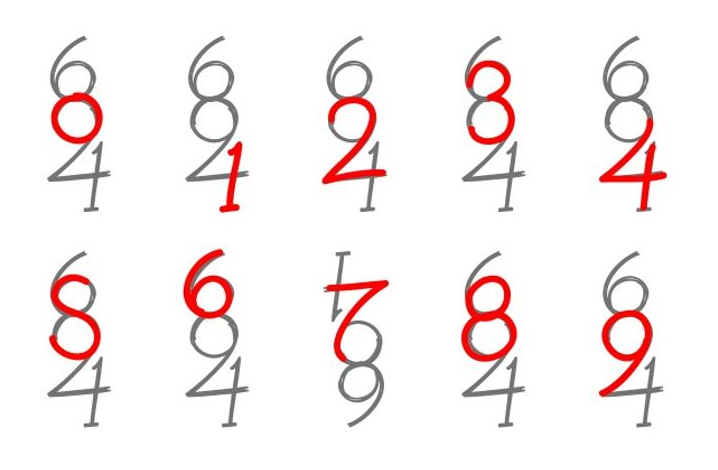source::

### STEM token GiveAway

I'll be again doing a giveaway of STEM tokens to the lucky random winner with the correct answer. For the last contest, which was How Many Numbers Do You See?.

We had 5 entries & none of them was correct. But; I'm still giving away the reward to @acont providing detailed information as to how he arrived with the 9 numbers. Congratulations @acont; You WIN yourself 10 STEM tokens. You should be having the rewards in your STEM Wallet Soon.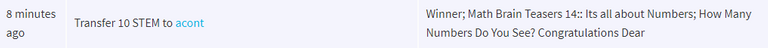### Math Quote for the Day::

Here is the motivation to solve this Number puzzle; How many numbers do you see??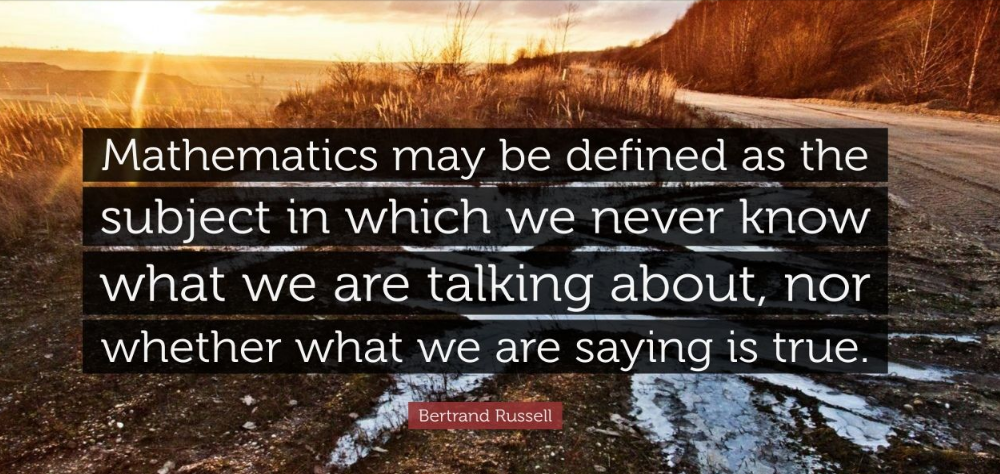source::

If you like my work, then please spread the Word.. that we do have the Math Brain Teasers competition here @StemGeeks platform. Reblog is much appreciated.

Best Regards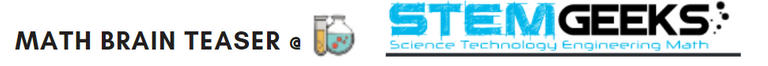Find Me on the Other Social Media Platforms::PS:: All the Maths Brain Teasers; are made by me using the Pro Canva License Version

Sort:

The answer is 21978. I figured out that only 2 and 8 are the numbers which when multiplied by 4 can give 8 and 2 which would be a reversible number. Then I just hit and trial the number D starting from 9 towards 0 and somehow after a long time, got the answer. That was a difficult one!!

2 years ago (edited)

Thanks for the rewards!

This one is hard, I'll keep thinking and maybe I'll leave my answer later. Greetings!